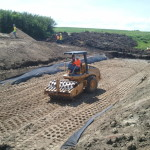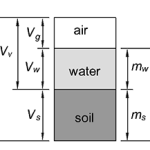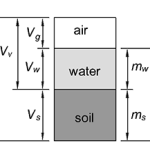## How to Calculate Relative DensityRelative Density is the ratio of the density of a substance to a specified reference density. Where: Dr = Relative Density (dimensionless) ρs = bulk density of soil (kg/m3 or lb/ft3) ρref = bulk density of reference (kg/m3 or lb/ft3) Often, the reference density is that of water, 62.4 lbs/ft3 or 1000 kg/m3. Alternatively, the […]

## How to Calculate Specific GravitySpecific Gravity is the ratio of the density of a soil to the density of water. Where: SG = Specific Gravity (dimensionless) ρs = bulk density of soil (kg/m3 or lb/ft3) ρw = bulk density of water (kg/m3 or lb/ft3) = 1000 kg/m3 (62.4 lb/ft3) Specific Gravity of Various Substances Substance Specific Gravity Water 1.0 […]

## How to Calculate Bulk DensityBulk density, also called soil density, is the ratio of the total mass of a soil to the total volume. Where: ρ = bulk density (kg/m3 or lb/ft3) mt = Total mass (kg or lbs) mw = Mass of water (kg or lbs) ms = Mass of solids (kg or lbs) Vt = Total Volume […]

## How to Calculate Degree of SaturationThe degree of saturation is the ratio of the volume of water in a soil to the volume of voids. Where: S = Degree of Saturation Vw = Volume of water (m3 or ft3) Vv = Volume of voids (m3 or ft3) Vg = Volume of air (m3 or ft3) The degree of saturation is […]

## How to Calculate Soil PorosityThe soil porosity, also called the void fraction, is the ratio of the volume of voids (open spaces, i.e. air and water) in a soil to the total volume. Where: Vv = Volume of voids (m3 or ft3) Vt = Total Volume (m3 or ft3) Vg = Volume of air (m3 or ft3) Vw = […]